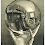## Monday, February 02, 2009

### Number is Out

Which of the following numbers is the odd one out?

3628, 2426, 4146, 1448

1.I don't feel that I have a good answer...
2426
- it is the only one that does not have 4 as a factor
- it is the only number whose digits sum to an even number

Or maybe its 3628, because its the only one containing an odd number. Even still it could be 4146, for its the only one whose prime factors are less than 100....

I fear I'm over-analyzing.

2.This might also be too complex,, but...

Construct the class of number such that:
-The second digit is the product of the first digit and the third digit (e.g. 3*2=6,2*2=4,1*4=4)
-The fourth digit is the sum of the third digit and the second digit (e.g. 6+2=8,2+4=6,4+4=8)

Then 4146 is the only number above not to fall in that class.

3.4146 -- the second digit is odd only in this number. First digit of each is either 1,2,3,4.
Third digit - 2,2,4,4
Fourth - 6,6,8,8

4.4146
In all the other numbers 1st digit is equal to the 2nd digit divided by the third and the last digit is the sum of second and third digit
3628 3=6/2 8=6+2
2426 2=4/2 6=4+2

1448 1=4/4 8=4+4

5.I can't believe anyone figured this one out. I really, really thought I would stump everyone.

The answer I was going for (the odd number argument is legitimate, just not the answer I was thinking of) was 4146

Let each number be ABCD.
Then A x C = B and B + C = D for three of the numbers.
For instance: 3628 = ABCD. Then 3 x 2 = 6 and 6 + 2 = 8.
The odd one out is 4146.

6.For all the numbers, the middle 2 digits add up to the last digit:
3628: 6 + 2 = 8
2426: 4 + 2 = 6
4146: 1 + 4 = 5 (not equal to 6)
1448: 4 + 4 = 8

So, 4146 is the odd one out!!

-Sayanti

Leave your answer or, if you want to post a question of your own, send me an e-mail. Look in the about section to find my e-mail address. If it's new, I'll post it soon.

Please don't leave spam or 'Awesome blog, come visit mine' messages. I'll delete them soon after.

Enter your Email and join hundreds of others who get their Question of the Day sent right to their mailbox

The Lamplight Manor Puzz 3-D